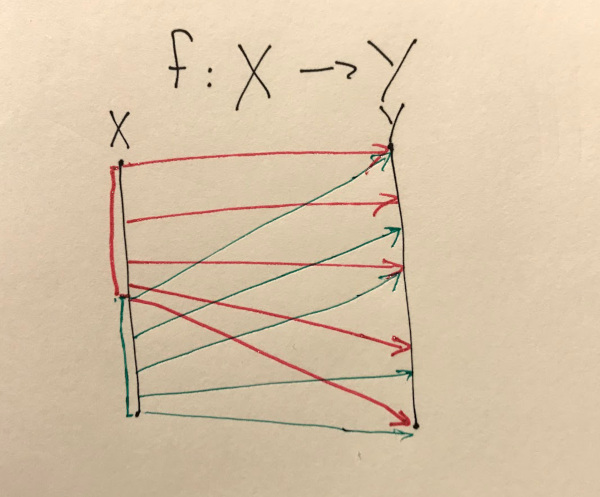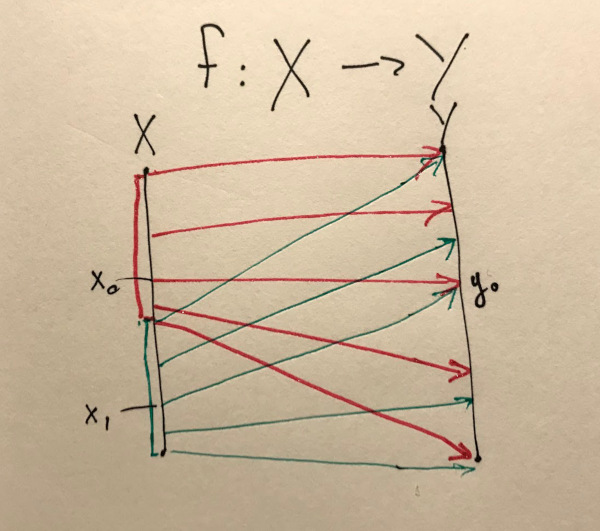# Singleton, Fiber and Equivalence

UPDATE: this article was posted on Groupoid Space, fonts are probably better there. Thanks, Maxim! http://groupoid.space/mltt/equiv/

I am reading a beautiful paper called "A self-contained, brief and complete formulation of Voevodsky’s Univalence Axiom" by Martín Hötzel Escardó, and it really takes time to understand some of the formulas. In fact, it takes so much time, that I've spent an hour on an airplane from Frankfurt to Kyiv gazing at just the first three of them. Intention of this article is not to create any new knowledge, but to extend available explanations with specific examples that helped me understand these formulas better, when they finally "clicked" this morning.

The formulas I am focusing on are Singleton, Fiber and Equivalence:

## Quick reminder on $\prod$ (Pi) and $\sum$ (Sigma) for programmers

Just a quick reminder that ${\prod}$ is read as "dependent function" (or "for all") and $\sum$ as "dependent tuple" ("there exist"). So, whenever you see:

you can mentally replace it with:

where <rest> can mention x in its type. And when you see:

you think

where <rest> can mention x in its type.

## Singleton

So, first question I had was: what is that isSingleton exactly? Is that a type? A function? A theorem?

Singleton can be understood easily in terms of its implementation in code. I'll use cubicaltt as the implementation language which is very minimal but powerful Haskell-like language and compiler. I'll make a type which has only one constructor and derive isSingleton for it.

data One = MkOne

Intuitively, One should be put instead of an X in the formula, so if we put it there, it becomes:

So, after we apply isSingleton to our type One, it becomes just a type for a dependent pair of this element and a function from any element of One to its equality to that element c. Or, in code, something like:

xxxxxxxxxxisSingletonOne = (c, \x -> <proof that x is equal to c>)

As can be seen, implementation consists of making a tuple of specific element c and a function proving its equality to any given x. Nice!

Due to cubicaltt readme, to make a Pi you just use syntax (x : A) -> B where B can refer to x, and for Sigma you use syntax (x : A) * B where * builds a tuple and B can refer to x in it. Full code looks like this:

​x-- Copied from prelude.ctt, just read Path as "equality of two elements of-- type A between a0 and a1", and refl is the way to make a PathPath (A : U) (a0 a1 : A) : U = PathP (<i> A) a0 a1refl (A : U) (a : A) : Path A a a = <i> a​IsSingleton (X : U) : U  = (c : X) * ((x : X) -> Path X c x)

Interestingly, IsSingleton returns a type, not a value. Well, values and types are all mixed in this dependent world, but it's important to get that a result of IsSingleton will be used in a type signature of our specialization for One. Let's use type holes to graduately derive our implementation:

xxxxxxxxxxIsSingletonOne : IsSingleton One  = ?

gives:

xxxxxxxxxxHole at (14,5) in singleton:​--------------------------------------------------------------------------------Sigma One (\(c : One) -> (x : One) -> PathP (<!0> One) c x)

Perfect! So, we've got our first answers:

• IsSingleton is sort of a type-level function, or type alias! You use it like IsSingleton YourType and get a type signature.
• It's also a theorem that you can prove by constructing a value, once you specialize it to some type, like One

We now need to choose c. Not a lot of options to choose from:

xxxxxxxxxxIsSingletonOne : IsSingleton One  = let c : One = MkOne    in (c, (\(x:One) -> ?))

This gives:

xxxxxxxxxxHole at (15,25) in singleton:​x : One--------------------------------------------------------------------------------PathP (<!0> One) MkOne x

Ok, now in order to implement that last ? we need to case-split. Case-splitting is best done at top-level in cubicaltt, so let's add a helper function and split there:

xxxxxxxxxxisSingletonOneInnerEq : (x:One) -> Path One MkOne x  = split  MkOne -> refl One MkOne​IsSingletonOne : IsSingleton One  = let c : One = MkOne    in (c, (\(x:One) -> isSingletonOneInnerEq x))

Allright! We've implemented a value of IsSingleton One, in other words, we've proven that One is a singleton type.

Full code can be seen at singleton.ctt

## Fiber

To understand a fiber and later equivalence best, I came up with a function where domain is split in two, and both halves are projected to full codomain range. Here's how it looks like:So, a Fiber would be a type, which, after some specific $y_0$ will be chosen, will look like this:

and to implement it, you'd need to provide a tuple of whatever x you like such that $f(x)$ will be equal to $y_0$.

In other words, both $x_0$ and $x_1$ would be ok as a first tuple's element:Fiber (X Y : U) (f : X -> Y) (y : Y) : U
= (x : X) * Path Y (f x) y


fiber.ctt

Implementation of specific fibers in cubicaltt is left to reader as an exercise :)

These are two fibers, both legit! But what is equivalence, now?

## Equivalence

The function f is called an equivalence if its fibers are all singletons

First, let's think for a moment, what does it mean that a fiber is a singleton or not?

Recall the formulas:

But now, instead of imagining $X$ to be some specific type, we need to imagine it to be a Fiber!

In other words, to prove that a fiber is singleton, I will give you a pair of some specific fiber, and a proof that for all fibers you give me in that point y, it will be equal to it.

Let's define the function we've seen on the drawing earlier like this:

halfsplit : (X Y : U) -> (X -> Y)
= undefined


You can imagine actual implementation by making real types for X and Y with some fixed set of points, but let's skip this for brevity.

Fiber for this function in some specific y (think $y_0$) would look like this:

halfsplitFiber : (X Y : U) (y : Y) -> Fiber X Y (halfsplit X Y) y
= undefined


So, what would it look like, to prove that this fiber is a singleton?

halfsplitFiberIsSingleton : (X Y : U) (y : Y)
-> IsSingleton (Fiber X Y (halfsplit X Y) y)
= undefined


Put IsSingleton definition in:

halfsplitFiberIsSingleton : (X Y : U) (y : Y)
-> (c : Fiber X Y (halfsplit X Y) y)
* ((x : Fiber X Y (halfsplit X Y) y)
-> Path (Fiber X Y (halfsplit X Y) y) c x)
= undefined


-- In order to prove that our halpsplit function's fiber is a singleton:
halfsplitFiberIsSingleton : (X Y : U) (y : Y)
-> (c : Fiber X Y (halfsplit X Y) y)
--   ^
--   \--- we need to provide some specific fiber in y0
* ((x : Fiber X Y (halfsplit X Y) y)
--     ^
--      \--- and a function, which, given any other fiber to y0
-> Path (Fiber X Y (halfsplit X Y) y) c x)
--      ^
--       \--- will prove that this given fiber is idential
--            to the one in tuple's fst element
= undefined


Now, think about the two fibers in question ($x_0 \to y_0$ and $x_1 \to y_0$), and you will understand, why this function will not have the Equivalence property:

The function f is called an equivalence if its fibers are all singletons

Thank you for your time. Please send your feedback in Issues or PRs in this blog's repo.